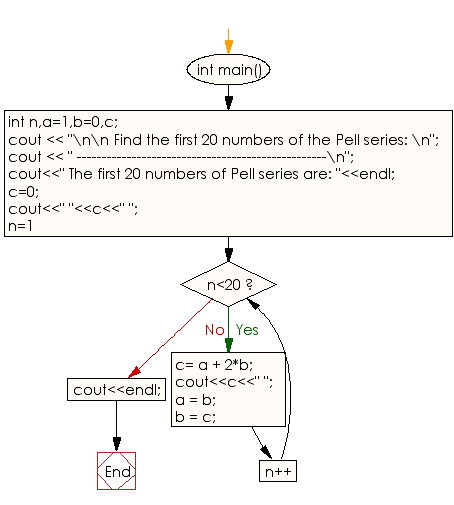﻿ C++ Exercises: Print the first 20 numbers of the Pell series - w3resource

# C++ Exercises: Print the first 20 numbers of the Pell series

## C++ Numbers: Exercise-39 with Solution

Write a C++ program to print the first 20 numbers of the Pell series.

Sample Solution:

C++ Code :

``````#include <iostream>
using namespace std;
int main()
{
int n,a=1,b=0,c;
cout << "\n\n Find the first 20 numbers of the Pell series: \n";
cout << " --------------------------------------------------\n";
cout<<" The first 20 numbers of Pell series are: "<<endl;
c=0;
cout<<" "<<c<<" ";
for(n=1; n<20; n++)
{
c= a + 2*b;
cout<<c<<" ";
a = b;
b = c;
}
cout<<endl;
}
``````

Sample Output:

```Find the first 20 numbers of the Pell series:
--------------------------------------------------
The first 20 numbers of Pell series are:
0 1 2 5 12 29 70 169 408 985 2378 5741 13860 33461 80782 195025 470832
1136689 2744210 6625109
```

Flowchart:C++ Code Editor:

What is the difficulty level of this exercise?

﻿

## C++ Programming: Tips of the Day

Why does the C++ map type argument require an empty constructor when using []?

This issue comes with operator[]. Quote from SGI documentation:

data_type&operator[](constkey_type& k) - Returns a reference to the object that is associated with a particular key. If the map does not already contain such an object, operator[] inserts the default object data_type().

If you don't have default constructor you can use insert/find functions. Following example works fine:

```myMap.insert(std::map<int, MyClass>::value_type ( 1, MyClass(1) ) );
myMap.find( 1 )->second;
```

Ref: https://bit.ly/3PQBRJi

We are closing our Disqus commenting system for some maintenanace issues. You may write to us at reach[at]yahoo[dot]com or visit us at Facebook